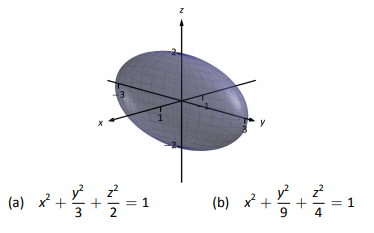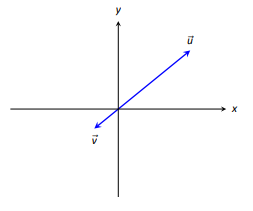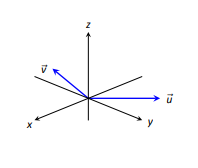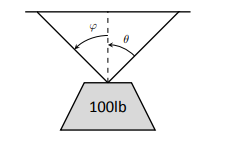$$\newcommand{\id}{\mathrm{id}}$$ $$\newcommand{\Span}{\mathrm{span}}$$ $$\newcommand{\kernel}{\mathrm{null}\,}$$ $$\newcommand{\range}{\mathrm{range}\,}$$ $$\newcommand{\RealPart}{\mathrm{Re}}$$ $$\newcommand{\ImaginaryPart}{\mathrm{Im}}$$ $$\newcommand{\Argument}{\mathrm{Arg}}$$ $$\newcommand{\norm}{\| #1 \|}$$ $$\newcommand{\inner}{\langle #1, #2 \rangle}$$ $$\newcommand{\Span}{\mathrm{span}}$$

# 10.E: Applications of Vectors (Exercises)

$$\newcommand{\vecs}{\overset { \rightharpoonup} {\mathbf{#1}} }$$ $$\newcommand{\vecd}{\overset{-\!-\!\rightharpoonup}{\vphantom{a}\smash {#1}}}$$$$\newcommand{\id}{\mathrm{id}}$$ $$\newcommand{\Span}{\mathrm{span}}$$ $$\newcommand{\kernel}{\mathrm{null}\,}$$ $$\newcommand{\range}{\mathrm{range}\,}$$ $$\newcommand{\RealPart}{\mathrm{Re}}$$ $$\newcommand{\ImaginaryPart}{\mathrm{Im}}$$ $$\newcommand{\Argument}{\mathrm{Arg}}$$ $$\newcommand{\norm}{\| #1 \|}$$ $$\newcommand{\inner}{\langle #1, #2 \rangle}$$ $$\newcommand{\Span}{\mathrm{span}}$$ $$\newcommand{\id}{\mathrm{id}}$$ $$\newcommand{\Span}{\mathrm{span}}$$ $$\newcommand{\kernel}{\mathrm{null}\,}$$ $$\newcommand{\range}{\mathrm{range}\,}$$ $$\newcommand{\RealPart}{\mathrm{Re}}$$ $$\newcommand{\ImaginaryPart}{\mathrm{Im}}$$ $$\newcommand{\Argument}{\mathrm{Arg}}$$ $$\newcommand{\norm}{\| #1 \|}$$ $$\newcommand{\inner}{\langle #1, #2 \rangle}$$ $$\newcommand{\Span}{\mathrm{span}}$$

## 10.1: Introduction to Cartesian Coordinates in Space

### Terms and Concepts

1. Axes drawn in space must conform to the ________ _________ rule.

2. In the plane, the equation $$x=2$$ defines a ________; in space, $$x=2$$ defines a ________.

3. In the plane, the equation $$y=x^2$$ defines a ________; in space, $$y=x^2$$ defines a ________.

4. Which quadric surface looks like a Pringles chip?

5. Consider the hyperbola $$x^2-y^2=1$$ in the plane. If this hyperbola is rotated about the x-axis, what quadric surface is formed?

6. Consider the hyperbola $$x^2-y^2=1$$ in the plane. If this hyperbola is rotated about the y-axis, what quadric surface is formed?

### Problems

7. The points $$A=(1,4,2),\,B=(2,6,3)\text{ and }C=(4,3,1)$$ form a triangle in space. Find the distances between each pair of points and determine if the triangle is a right triangle.

8. The points $$A=(1,1,3),\,B=(3,2,7),\,C=(2,0,8)\text{ and }D=(0,-1,4)$$ form a quadrilateral ABCD in space. Is this a parallelogram?

9. Find the center and radius of the sphere defined by $$x^2-8x+y^2+2y+z^2+8=0$$.

10. Find the center and radius of the sphere defined by $$x^2+y^2+z^2+4x-2y-4z+4=0$$.

In Exercises 11-14, describe the region in space defined by the inequalities.

11. $$x^2+y^2+z^2 <1$$

12. $$0\le x \le 3$$

13. $$x\ge 0, y\ge 0, z\ge 0$$

14. $$y\ge 3$$

In Exercises 15-18, sketch the cylinder in space.

15. $$z=x^3$$

16. $$y=\cos z$$

17. $$\frac{x^2}{4}+\frac{y^2}{9}=1$$

18. $$y=\frac{1}{x}$$

In Exercises 19-22, give the equation of the surface of revolution described.

19. Revolve $$z=\frac{1}{1+y^2}$$ about the y-axis.

20. Revolve $$y=x^2$$ about the x-axis.

21. Revolve $$z=x^2$$ about the z-axis.

22. Revolve $$z=1/x$$ about the z-axis.

In Exercises 23-26, a quadric surface is sketched. Determine which of the given equations best fits the graph.

23.24.25.26.In Exercises 27-32, sketch the quadric surface.

27. $$z-y^2+x^2=0$$

28. $$z^2=x^2+\frac{y^2}{4}$$

29. $$x=-y^2-z^2$$

30. $$16x^2-16y^2-16z^2=1$$

31. $$\frac{x^2}{9}-y^2+\frac{z^2}{25}=1$$

32. $$4x^2+2y^2+z^2=4$$

## 10.2: An Introduction to Vectors

### Terms and Concepts

1. Name two different things that cannot be described with just one number, but rather need 2 or more numbers to fully describe them.

2. What is the difference between $$(1,2)\text{ and }\langle 1,2\rangle$$?

3. What is a unit vector?

4. What does it mean for two vectors to be parallel?

5. What effect does multiplying by a vector by -2 have?

### Problems

In Exercises 6-9, points P and Q are given. Write the vector $$\vec{PQ}$$ in component form and using the standard unit vectors.

6. $$P=(2,-1),\quad Q=(3,5)$$

7. $$P=(3,2),\quad Q=(7,-2)$$

8. $$P=(0,3,-1),\quad Q=(6,2,5)$$

9. $$P=(2,1,2),\quad Q=(4,3,2)$$

10. Let $$\vec{u}=\langle 1,-2 \rangle \text{ and }\vec{v} =\langle 1,1 \rangle$$.
(a) Find $$\vec{u}+\vec{v},\,\vec{u}-\vec{v},\,2\vec{u}-3\vec{v}$$.
(b) Sketch the above vectors on the same axes, along with $$\vec{u}\text{ and }\vec{v}$$.
(c) Find $$\vec{x}$$ where $$\vec{u}+\vec{x}=2\vec{v}-\vec{x}$$.

11. Let $$\vec{u}=\langle 1,1,-1 \rangle \text{ and }\vec{v}=\langle 2,1,2 \rangle$$.
(a) Find $$\vec{u}+\vec{v},\,\vec{u}-\vec{v},\,\pi \vec{u}-\sqrt{2}\vec{v}$$.
(b) Sketch the above vectors on the same axes, along with $$\vec{u}\text{ and }\vec{v}$$.
(c) Find $$\vec{x}$$ where $$\vec{u}+\vec{x}=\vec{v}+2\vec{x}$$.

In Exercises 12-15, sketch $$\vec{u},\vec{v},\vec{u}+\vec{v} \text{ and }\vec{u}-\vec{v}$$ on the same axes.

12.13.14.15.In Exercises 16-19, find $$\lVert \vec{u}\rVert,\lVert \vec{v}\rVert,\lVert \vec{u}+\vec{v}\rVert \text{ and }\lVert \vec{u}-\vec{v}\rVert$$.

16. $$\vec{u}=\langle 2,1 \rangle,\quad \vec{v}=\langle 3,-2\rangle$$

17. $$\vec{u}=\langle -3,2,2 \rangle,\quad \vec{v}=\langle 1,-1,1\rangle$$

18. $$\vec{u}=\langle 1,2 \rangle,\quad \vec{v}=\langle -3,-6\rangle$$

19. $$\vec{u}=\langle 2,-3,6 \rangle,\quad \vec{v}=\langle 10,-15,30\rangle$$

20. Under what conditions is $$\lVert \vec{u}\rVert+\lVert \vec{v}\rVert=\lVert \vec{u}+\vec{v} \rVert$$?

In Exercises 21-24, find the unit vector $$\vec{u}$$ in the direction of $$\vec{v}$$.

21. $$\vec{v} = \langle 3,7 \rangle$$

22. $$\vec{v} = \langle 6,8 \rangle$$

23. $$\vec{v} = \langle 1,-2,2 \rangle$$

24. $$\vec{v} = \langle 2,-2,2 \rangle$$

25. Find the unit vector in the first quadrant of $$\mathbb{R}^2$$ that makes a $$50^\circ$$ angle with the x-axis.

26. Find the unit vector in the second quadrant of $$\mathbb{R}^2$$ that makes a $$30^\circ$$ angle with the y-axis.

27. Verify, from Key Idea 48, that $$\vec{u}=\langle \sin \theta \cos \phi ,\sin \theta \sin \phi, \cos \theta \rangle$$ is a unit vector for all angles $$\theta \text{ and }\phi$$.

A weight of 100lb is suspended from two chains, making an angles with the vertical of $$\theta\text{ and }\phi$$ as shown in the figure below.In Exercises 28-31, angles $$\theta \text{ and }\phi$$ are given. Find the force applied to each chain.

28. $$\theta =30^\circ,\quad \phi=30^\circ$$

29. $$\theta =60^\circ,\quad \phi=60^\circ$$

30. $$\theta =20^\circ,\quad \phi=15^\circ$$

31. $$\theta =0^\circ,\quad \phi=0^\circ$$

A weight of $$p$$lb is suspended from a chain of length $$l$$ while a constant force of $$\vec{F}_w$$ pushes the weight to the right, making an angle of $$\theta$$ with the vertical, as shown in the figure below.In Exercises 32-35, a force $$\vec{F}_w$$ and length $$l$$ are given. Find the angle $$\theta$$ and the height the weight is lifted as it moves to the right.

32. $$\vec{F}_w=1\text{lb},\quad l=1\text{ft},\quad p=1\text{lb}$$

33. $$\vec{F}_w=1\text{lb},\quad l=1\text{ft},\quad p=10\text{lb}$$

34. $$\vec{F}_w=1\text{lb},\quad l=10\text{ft},\quad p=1\text{lb}$$

35. $$\vec{F}_w=10\text{lb},\quad l=10\text{ft},\quad p=1\text{lb}$$

## 10.3: The Dot Product

### Terms and Concepts

1. The dot product of two vectors is a __________, not a vector.

2. How are the concepts of the dot product and vector magnitude related?

3. How can one quickly tell if the angle between two vectors is acute or obtuse?

4. Give a synonym for "orthogonal."

### Problems

In Exercises 5-10, find the dot product of the given vectors.

5. $$\vec{u}=\langle 2,-4 \rangle, \vec{v}=\langle 3,7 \rangle$$

6. $$\vec{u}=\langle 5,3 \rangle, \vec{v}=\langle 6,1 \rangle$$

7. $$\vec{u}=\langle 1,-1,2 \rangle, \vec{v}=\langle 2,5,3 \rangle$$

8. $$\vec{u}=\langle 3,5,-1 \rangle, \vec{v}=\langle 4,-1,7 \rangle$$

9. $$\vec{u}=\langle 1,1 \rangle, \vec{v}=\langle 1,2,3 \rangle$$

10. $$\vec{u}=\langle 1,2,3 \rangle, \vec{v}=\langle 0,0,0 \rangle$$

11. Create your own vectors $$\vec{u},\vec{v},\text{ and }\vec{w}\text{ in }\mathbb{R}^2$$ and show that $$\vec{u}\cdot (\vec{v}+\vec{w})=\vec{u}\cdot \vec{v}+\vec{u}\cdot \vec{w}$$.

12. Create your own vectors $$\vec{u}\text{ and }\vec{v}\text{ in }\mathbb{R}^3$$ and scalar c and show that $$c(\vec{u}\cdot \vec{v})=\vec{u}\cdot (c\vec{v})$$.

In Exercises 13-16, find the measure of the angle between the two vectors in both radians and degrees.

13. $$\vec{u}=\langle 1,1 \rangle,\,\vec{v}=\langle 1,2 \rangle$$

14. $$\vec{u}=\langle -2,1 \rangle,\,\vec{v}=\langle 3,5 \rangle$$

15. $$\vec{u}=\langle 8,1,-4 \rangle,\,\vec{v}=\langle 2,2,0 \rangle$$

16. $$\vec{u}=\langle 1,7,2 \rangle,\,\vec{v}=\langle 4,-2,5 \rangle$$

In Exercises 17-20, a vectors $$\vec{v}$$ is given. Give two vectors that are orthogonal to $$\vec{v}$$.

17. $$\vec{v}=\langle 4,7 \rangle$$

18. $$\vec{v}=\langle -3,5 \rangle$$

19. $$\vec{v}=\langle 1,1,1 \rangle$$

20. $$\vec{v}=\langle 1,-2,3 \rangle$$

In Exercises 21-26, vectors $$\vec{u}\text{ and }\vec{v}$$ are given. Find $$\text{proj}_{\vec{v}}\vec{u}$$, the orthogonal projection of $$\vec{u}$$ onto $$\vec{v}$$, and sketch all three vectors on the same axes.

21. $$\vec{u}=\langle 1,2 \rangle , \vec{v}=\langle -1,3 \rangle$$

22. $$\vec{u}=\langle 5,5 \rangle , \vec{v}=\langle 1,3 \rangle$$

23. $$\vec{u}=\langle -3,2 \rangle , \vec{v}=\langle 1,1 \rangle$$

24. $$\vec{u}=\langle -3,2 \rangle , \vec{v}=\langle 2,3 \rangle$$

25. $$\vec{u}=\langle 1,5,1 \rangle , \vec{v}=\langle 1,2,3 \rangle$$

26. $$\vec{u}=\langle 3,-1,2 \rangle , \vec{v}=\langle 2,2,1 \rangle$$

In Exercises 27-32, vectors $$\vec{u}$$ and $$vec{v}$$ are given. Write $$\vec{u}$$ as the sum of two vectors, one of which is parallel to $$\vec{v}$$ and one of which is perpendicular to $$\vec{v}$$. Note: these are the same pairs of vectors as found in Exercises 21-26.

27. $$\vec{u}=\langle 1,2 \rangle , \vec{v}=\langle -1,3 \rangle$$

28. $$\vec{u}=\langle 5,5 \rangle , \vec{v}=\langle 1,3 \rangle$$

29. $$\vec{u}=\langle -3,2 \rangle , \vec{v}=\langle 1,1 \rangle$$

30. $$\vec{u}=\langle -3,2 \rangle , \vec{v}=\langle 2,3 \rangle$$

31. $$\vec{u}=\langle 1,5,1 \rangle , \vec{v}=\langle 1,2,3 \rangle$$

32. $$\vec{u}=\langle 3,-1,2 \rangle , \vec{v}=\langle 2,2,1 \rangle$$

33. A 10lb box sits on a ramp that rises 4ft over a distance of 20ft. How much force is required to keep the box from sliding down the ramp?

34. A 10lb box sits on a 15ft ramp that makes a 30$$^\circ$$ angle with the horizontal. How much force is required to keep the box from sliding down the ramp?

35. How much work is performed in moving a box horizontally 10ft with a force of 20lb applied at an angle of 45$$^\circ$$ to the horizontal?

36. How much work is performed in moving a box horizontally 10ft with a force of 20lb applied at an angle of 10$$^\circ$$ to the horizontal?

37. How much work is performed in moving a box up the length of a ramp that rises 2ft over a distance of 10ft, with a force of 50lb applied horizontally?

38. How much work is performed in moving a box up the length of a ramp that rises 2ft over a distance of 10ft, with a force of 50lb applied at an angle of 45$$^\circ$$ to the horizontal?

39. How much work is performed in moving a box up the length of a 10ft ramp that makes a 5$$^\circ$$ angle with the horizontal, with 50lb of force applied in the direction of the ramp?

## 10.4: The Cross Product

### Terms and Concepts

1. The cross product of two vectors is a ________, not a scalar.

2. One can visualize the direction of $$\vec{u}\times \vec{v}$$ using the _______ ________ ________.

3. Give synonym for "orthogonal."

4. T/F: A fundamental principle of the cross product is that $$\vec{u}\times\vec{v}$$ is orthogonal to $$\vec{u}$$ and $$\vec{v}$$.

5. _______ is a measure of the turning force applied to an object.

### Problems

In Exercises 6-14, vectors $$\vec{u}$$ and $$\vec{v}$$ are given. Compute $$\vec{u}\times \vec{v}$$ and show this is orthogonal to both $$\vec{u}$$ and $$\vec{v}$$.

6. $$\vec{u}=\langle 3,2,-2 \rangle ,\, \vec{v}=\langle 0,1,5 \rangle$$

7. $$\vec{u}=\langle 5,-4,3 \rangle ,\, \vec{v}=\langle 2,-5,1 \rangle$$

8. $$\vec{u}=\langle 4,-5,-5 \rangle ,\, \vec{v}=\langle 3,3,4 \rangle$$

9. $$\vec{u}=\langle -4,7,-10 \rangle ,\, \vec{v}=\langle 4,4,1 \rangle$$

10. $$\vec{u}=\langle 1,0,1 \rangle ,\, \vec{v}=\langle 5,0,7 \rangle$$

11. $$\vec{u}=\langle 1,5,-4 \rangle ,\, \vec{v}=\langle -2,-10,8 \rangle$$

12. $$\vec{u}=\vec{i} ,\, \vec{v}=\vec{j}$$

13. $$\vec{u}=\vec{i} ,\, \vec{v}=\vec{k}$$

14. $$\vec{u}=\vec{j} ,\, \vec{v}=\vec{j}$$

15. Pick any vectors $$\vec{u},\vec{v}$$ and $$\vec{w}$$ in $$\mathbb{R}^3$$ and show that $$\vec{u}\times (\vec{v}+\vec{w}=\vec{u}\times \vec{v} +\vec{u}\times \vec{w}$$.

16. Pick any vectors $$\vec{u},\vec{v}$$ and $$\vec{w}$$ in $$\mathbb{R}^3$$ and show that $$\vec{u}\cdot (\vec{v}\times\vec{w}=(\vec{u}\times \vec{v}) \cdot \vec{w}$$.

In Exercises 17-20, the magnitude of vectors $$\vec{u}$$ and $$\vec{v}$$ in $$\mathbb{R}^3$$ are given, along with the angle $$\theta$$ between them. Use this information to find the magnitude of $$\vec{u}\times \vec{v}$$.

17. $$\lVert \vec{u} \rVert=2 , \quad \lVert \vec{v}\rVert = 5,\quad \theta =30^\circ$$

18. $$\lVert \vec{u} \rVert=3 , \quad \lVert \vec{v}\rVert = 7,\quad \theta =\pi/2$$

19. $$\lVert \vec{u} \rVert=3 , \quad \lVert \vec{v}\rVert = 4,\quad \theta =\pi$$

20. $$\lVert \vec{u} \rVert=2 , \quad \lVert \vec{v}\rVert = 5,\quad \theta =5\pi /6$$

In Exercises 21-24, find the area of the parallelogram defined by the given vectors.

21. $$\vec{u}=\langle 1,1,2 \rangle ,\, \vec{v}=\langle 2,0,3 \rangle$$

22. $$\vec{u}=\langle -2,1,5 \rangle ,\, \vec{v}=\langle -1,3,1 \rangle$$

23. $$\vec{u}=\langle 1,2 \rangle ,\, \vec{v}=\langle 2,1 \rangle$$

24. $$\vec{u}=\langle 2,0 \rangle ,\, \vec{v}=\langle 0,3 \rangle$$

In Exercises 25-28, find the area of the triangle with the given vertices.

25. Vertices: $$(0,0,0), (1,3,-1)\text{ and }(2,1,1)$$.

26. Vertices: $$(5,2,-1), (3,6,2)\text{ and }(1,0,4)$$.

27. Vertices: $$(1,1), (1,3)\text{ and }(2,2)$$.

28. Vertices: $$(3,1), (1,2)\text{ and }(4,3)$$.

In Exercises 29-30, find the area of the quadrilateral with the given vertices. (Hint: break the quadrilateral into the 2 triangles.)

29. Vertices: $$(0,0),(1,2),(3,0)\text{ and }(4,3)$$.

30. Vertices: $$(0,0,0),(2,1,1),(-1,2,-8)\text{ and }(1,-1,5)$$.

In Exercises 31-32, find the volume of the parallelepiped defined by the given vectors.

31. $$\vec{u}=\langle 1,1,1 \rangle,\quad \vec{v}=\langle 1,2,3 \rangle,\quad \vec{w}=\langle 1,0,1 \rangle$$

32. $$\vec{u}=\langle -1,2,1 \rangle,\quad \vec{v}=\langle 2,2,1 \rangle,\quad \vec{w}=\langle 3,1,3 \rangle$$

In Exercises 33-36, find a unit vector orthogonal to both $$\vec{u}$$ and $$\vec{v}$$.

33. $$\vec{u}=\langle 1,1,1 \rangle ,\quad \vec{v}=\langle 2,0,1 \rangle$$

34. $$\vec{u}=\langle 1,-2,1 \rangle ,\quad \vec{v}=\langle 3,2,1 \rangle$$

35. $$\vec{u}=\langle 5,0,2 \rangle ,\quad \vec{v}=\langle -3,0,7 \rangle$$

36. $$\vec{u}=\langle 1,-2,1 \rangle ,\quad \vec{v}=\langle -2,4,-2 \rangle$$

37. A bicycle rider applies 150lb of force, straight down, onto a pedal that extends ϳin horizontally from the crankshaft. Find the magnitude of the torque applied to the crankshaft.

38. A bicycle rider applies 150lb of force, straight down, onto a pedal that extends 7in from the crankshaft, making a 30$$^\circ$$ angle with the horizontal. Find the magnitude of the torque applied to the crankshaft.

39. To turn a stubborn bolt, 80lb of force is applied to a 10in wrench. What is the maximum amount of torque that can be applied to the bolt?

40. To turn a stubborn bolt, 80lb of force is applied to a 10in wrench in a confined space, where the direction of applied force makes a 10$$^\circ$$ angle with the wrench. How much torque is subsequently applied to the wrench?

41. Show, using the definition of the Cross Product, that $$\vec{u}\cdot (\vec{u}\times \vec{v})=0$$; that is, that $$\vec{u}$$ is orthogonal to the cross product of $$\vec{u}$$ and $$\vec{v}$$.

42. Show, using the definition of the Cross Product, that $$vec{u}\times\vec{u}=\vec{0}$$.

## 10.5: Lines

### Terms and Concepts

1. To find an equation of a line, what two pieces of information are needed?

2. Two distinct lines in the plane can intersect or be _______.

3. Two distinct lines in space can intersect, be _________ or be __________.

4. Use your own words to describe what it means for two lines in space to be skew.

### Problems

In Exercises 5-14, write the vector, parametric and symmetric equations of the lines described.

5. Passes through $$P=(2,-4,1)$$, parallel to $$\vec{d}=\langle 9,2,5\rangle$$.

6. Passes through $$P=(6,1,7)$$, parallel to $$\vec{d}=\langle -3,2,5 \rangle$$.

7. Passes through $$P=(2,1,5)$$ and $$Q=(7,-2,4)$$.

8. Passes through $$P=(1,-2,3)$$ and $$Q=(5,5,5)$$.

9. Passes through $$P=(0,1,2)$$ and orthogonal to both $$\vec{d}_1 = \langle 2,-1,7 \rangle$$ and $$\vec{d}_2 = \langle 7,1,3 \rangle$$.

10. Passes through $$P=(5,1,9)$$ and orthogonal to both $$\vec{d}_1 = \langle 1,0,1 \rangle$$ and $$\vec{d}_2 = \langle 2,0,3\rangle$$.

11. Passes through the point of intersection of $$\vec{l}_1 (t)$$ and $$\vec{l}_2 (t)$$ and orthogonal to both lines, where
$$\vec{l}_1 (t) = \langle 2,1,1 \rangle + t\langle 5,1,-2 \rangle$$ and
$$\vec{l}_2 (t) = \langle -2,-1,2 \rangle + t\langle 3,1,-1 \rangle$$.

12. Passes through the point of intersection of $$\vec{l}_1 (t)\text{ and }\vec{l}_2 (t)$$ and orthogonal to both lines, where
$$l_1 = \begin{cases} x=t \\ y=-2+2t \\ z=1+t \end{cases}\text{ and }l_2 = \begin{cases}x=2+t \\ y=2-t \\ z=1+t \end{cases}$$

13. Passes through $$P=(1,1)$$, parallel to $$\vec{d}=\langle 2,3 \rangle$$.

14. Passes through $$P=(-2,5)$$, parallel to $$\vec{d}=\langle 0,1 \rangle$$.

In Exercises 15-22, determine if the described lines are the same line, parallel lines, intersecting or skew lines. If intersecting, give the point of intersection.

15.
$$\vec{l}_1(t) = \langle 1,2,1 \rangle +t\langle 2,-1,1 \rangle , \vec{l}_2(t) = \langle 3,3,3 \rangle +t\langle -4,2,-2 \rangle$$.

16.
$$\vec{l}_1(t) = \langle 2,1,1 \rangle +t\langle 5,1,3 \rangle , \vec{l}_2(t) = \langle 14,5,9 \rangle +t\langle 1,1,1 \rangle$$.

17.
$$\vec{l}_1(t) = \langle 3,4,1 \rangle +t\langle 2,-3,4 \rangle , \vec{l}_2(t) = \langle -3,3,-3 \rangle +t\langle 3,-2,4 \rangle$$.

18.
$$\vec{l}_1(t) = \langle 1,1,1 \rangle +t\langle 3,1,3 \rangle , \vec{l}_2(t) = \langle 7,3,7 \rangle +t\langle 6,2,6 \rangle$$.

19.
$$l_1 = \begin{cases}x=1+2t \\ y=3-2t \\ z=t \end{cases}\text{ and }l_2 = \begin{cases}x=3-t \\ y=2+5t \\ z=2+7t \end{cases}$$

20.
$$l_1 = \begin{cases}x=1.1+0.6t \\ y=3.77+0.9t \\ z=-2.3+1.5t \end{cases}\text{ and }l_2 = \begin{cases}x=3.11+3.4t \\ y=2+5.1t \\ z=2.5+8.5t \end{cases}$$

21.
$$l_1 = \begin{cases}x=-0.2+0.6t \\ y=1.33-0.45t \\ z=-4.2+1.05t \end{cases}\text{ and }l_2 = \begin{cases}x=0.86+9.2t \\ y=0.835+9.2t \\ z=-3.045+16.1t\end{cases}$$

22.
$$l_1 = \begin{cases}x=0.1+1.1t \\ y=2.9-1.5t \\ z=3.2+1.6t \end{cases}\text{ and }l_2 = \begin{cases}x=4-2.1t \\ y=1.8+7.2t \\ z=3.1+1.1t \end{cases}$$

In Exercises 23-26, find the distance from the point to the line.

23. $$P=(1,1,1),\quad \vec{l}(t) = \langle 2,1,3 \rangle +t\langle 2,1,-2 \rangle$$

24. $$P=(2,5,6),\quad \vec{l}(t) = \langle -1,1,1 \rangle +t\langle 1,0,1 \rangle$$

25. $$P=(0,3),\quad \vec{l}(t) = \langle 2,0 \rangle +t\langle 1,1 \rangle$$

26. $$P=(1,1),\quad \vec{l}(t) = \langle 4,5 \rangle +t\langle -4,3 \rangle$$

In Exercises 27-28, find the distance between the two lines.

27.
$$\vec{l}_1(t) = \langle 1,2,1 \rangle +t\langle 2,-1,1 \rangle , \vec{l}_2(t) = \langle 3,3,3 \rangle +t\langle 4,2,-2 \rangle$$.

28.
$$\vec{l}_1(t) = \langle 0,0,1 \rangle +t\langle 1,0,0 \rangle , \vec{l}_2(t) = \langle 0,0,3 \rangle +t\langle 0,1,0 \rangle$$.

Exercises 29-31 explore special cases of the distance formulas found in Key Idea 50.

29. Let $$Q$$ be a point on the line $$l(t)$$. Show why the distance formula correctly gives the distance from the point to the line as 0.

30. Let lines $$l_1(t)\text{ and } l_2(t)$$ be intersecting lines. Show why the distance formula correctly gives the distance between these lines as 0.

31. Let lines $$l_1(t)\text{ and }l_2(t)$$ be parallel.
(a) Show why the distance formula for distance between lines cannot be used as stated to find the distance between the lines.
(b) Show why letting $$c=(\vec{P_1P_2}\times \vec{d_2})\times \vec{d_2}$$ allows one to the use the formula.
(c) Show how one can use the formula for the distance between a point and a line and a line to find the distance between parallel lines.

## 10.6: Planes

### Terms and Concepts

1. In order to find the equation of a plane, what two pieces of information must one have?

2. What is the relationship between a plane and one of its normal vectors?

### Problems

In Exercises 3-6, give any two points in the given plane.

3. $$2x-4y+7z=2$$

4. $$3(x+2)+5(y-9)-4z=0$$

5. $$x=2$$

6. $$4(y+2)-(z-6)=0$$

In Exercises 7-20, give the equation of the described plane in standard and general forms.

7. Passes through $$(2,3,4)$$ and has normal vector $$\vec{n}=\langle 3,-1,7\rangle$$.

8. Passes through $$(1,3,5)$$ and has normal vector $$\vec{n}=\langle 0,2,4\rangle$$.

9. Passes through the points $$(1,2,3),(3,-1,4)\text{ and }(1,0,1)$$.

10. Passes through the points $$(5,3,8),(6,4,9)\text{ and }(3,3,3)$$.

11. Contains the intersecting lines
$$l_1(t) = \langle 2,1,2 \rangle +t\langle 1,2,3 \rangle$$ and
$$l_2(t) = \langle 2,1,2 \rangle +t\langle 2,5,4 \rangle$$.

12. Contains the intersecting lines
$$l_1(t) = \langle 5,0,3 \rangle +t\langle -1,1,1 \rangle$$ and
$$l_2(t) = \langle 1,4,7 \rangle +t\langle 3,0,-3 \rangle$$.

13. Contains the parallel lines
$$l_1(t) = \langle 1,1,1 \rangle +t\langle 1,2,3 \rangle$$ and
$$l_2(t) = \langle 1,1,2 \rangle +t\langle 1,2,3 \rangle$$.

14. Contains the parallel lines
$$l_1(t) = \langle 2,1,2 \rangle +t\langle 1,2,3 \rangle$$ and
$$l_2(t) = \langle 2,1,2 \rangle +t\langle 2,5,4 \rangle$$.

15. Contains the point $$(2,-6,1)$$ and the line
$$l(t) = \begin{cases}x=2+5t \\ y=2+2t \\ z=-1+2t \end{cases}$$

16. Contains the point $$(5,7,3)$$ and the line
$$l(t) = \begin{cases}x=t \\ y=t \\ z=t \end{cases}$$

17. Contains the point $$(5,7,3)$$ and is orthogonal to the line $$l(t) = \langle 4,5,6 \rangle +t\langle 1,1,1 \rangle$$.

18. Contains the point $$(4,1,1)$$ and is orthogonal to the line
$$l(t) = \begin{cases}x=4+4t \\ y=1+1t \\ z=1+1t \end{cases}$$

19. Contains the point $$(-4,7,2)$$ and is parallel to the plane $$3(x-2)+8(y+1)-10z=0$$.

20. Contains the point $$(1,2,3)$$ and is parallel to the plane $$x=5$$.

In Exercises 21-22, give the equation of the line that is the intersection of the given plane.

21.
$$p1: \, 3(x-2)+(y-1)+4z=0,\text{ and }$$
$$p2:\,2(x-1)-2(y+3)+6(z-1)=0$$.

22.
$$p1: \, 5(x-5)+2(y+2)+4(z-1)=0,\text{ and }$$
$$p2:\,3x-4(y-1)+2(z-1)=0$$.

In Exercises 23-26, find the point of intersection between the line and the plane.

23.
line: $$\langle 5,1,-1 \rangle +t\langle 2,2,1 \rangle ,$$
plane: $$5x-y-z=-3$$

24.
line: $$\langle 4,1,0 \rangle +t\langle 1,0,-1 \rangle ,$$
plane: $$3x+y-2x=8$$

25.
line: $$\langle 1,2,3 \rangle +t\langle 3,5,-1 \rangle ,$$
​​​​​​​plane: $$3x-2y-z=4$$

26.
line: $$\langle 1,2,3 \rangle +t\langle 3,5,-1 \rangle ,$$
​​​​​​​plane: $$3x-2y-z=-4$$

In Exercises 27-30, find the given distances.

27. The distance from the point $$(1,2,3)$$ to the plane
$$3(x-1)+(y-2)+5(z-2)=0$$.

28. The distance from the point $$(2,6,2)$$ to the plane
​​​​​​​$$2(x-1)-y+4(z+1)=0$$.

29. The distance between the parallel planes
$$x+y+z=0\text{ and }$$
$$(x-2)+(y-3)+(z+4)=0$$.

30. The distance between parallel planes
$$2(x-1)+2(y+1)+(z-2)=0\text{ and}$$
$$2(x-3)+2(y-1)+(z-3)=0$$

31. Show why if the point Q lies in a plane, then the distance formula correctly gives the distance from the point to the plane as 0.

32. How is Exercise 30 in Section 10.5 easier to answer once we have an understanding of planes?